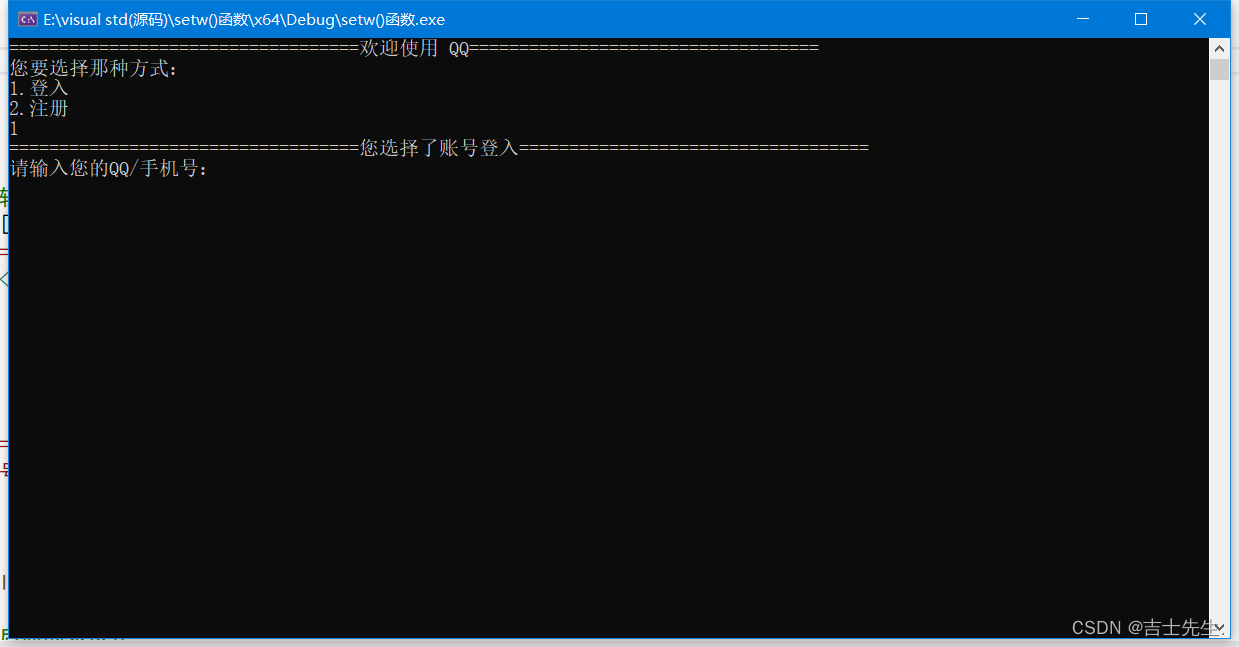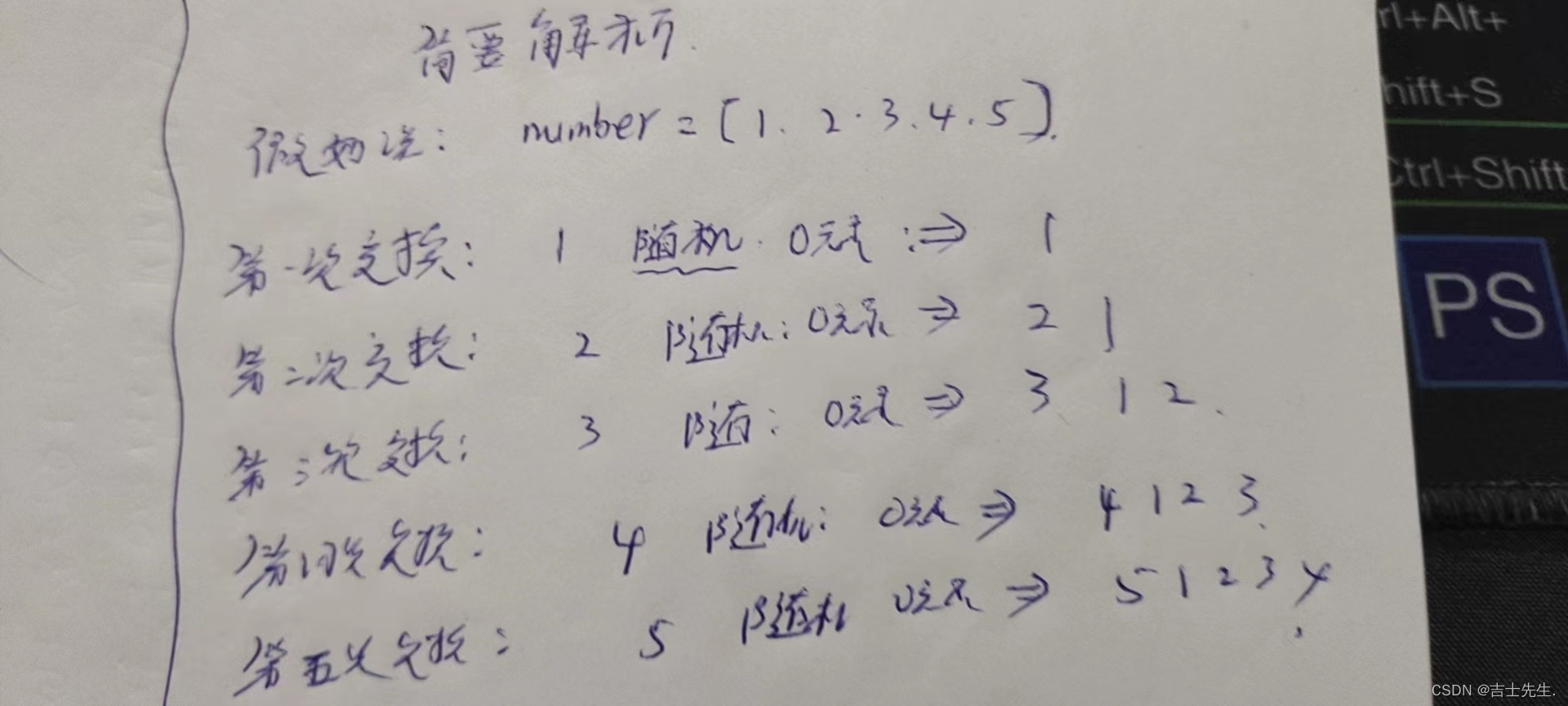# 43.【Java 实现验证码获取 C++实现密码加密和删除和QQ登入系统】

+关注继续查看

## (一)、简要概述:

### 1.思路分析

import java.util.Random
Random r=new Random()
r.netxtInt();

### 2.代码展示:

package test;  //  包名
import java.util.Random;
public class test1 {     //项目名
static int count=48;      //目的是为了 数字0到9
public static void main(String []avgs)
{
Random r=new Random();       //随机数声明
char Eng[]=new char;
for(int i=0;i<Eng.length;i++)
{
if(i<=25)             //得出来小写字母
{
Eng[i]=(char) (97+i);
}
else if(i>25&&i<52)      //得出来大写字母
{
Eng[i]=(char)(65+i-26)  ;//27
}
else                   //得出来阿拉侬数字
{
Eng[i]=(char)(count);
count++;
}
}
String s=" ";             //进行合并复制
for(int i=0;i<6;i++)
{
int EngRandomIdex=r.nextInt(Eng.length);
s=s+Eng[EngRandomIdex];
}
System.out.println("验证码是:"+s);       //输出

/*  for(int i=0;i<Eng.length;i++)
{
System.out.println(Eng[i]+" ");
}*/
}
}

## （二）、密码加密C++操作

### 2.代码展示：

#include <iostream>
#include <conio.h>
#include <string.h>
using namespace std;
int main()
{
char s;
string s1 = "*";
char c;
int i = 0;
cout << "请输入您的密码:" << endl;
while (1)
{
c = _getch();
if (c == '\r')break;   //按下enter就会退出
if (c == '\b')   //按下backspace键就会删除
{
if (i > 0)        //目的是删除储存进来的那个数组元素
{
i--;
}
printf("\b");  //回退一格光标
printf(" ");    //打印一个空格把要删除的字符覆盖了
printf("\b");   //再回退一格光标来掩饰掉打印的空格
continue;      //不能省略，一旦省略就会出现删除不动的错误.
}
s[i++] = c;
cout << "*";
}
cout << "您输入的密码是:" ;
for (int j = 0; j < i; j++)
{
cout << s[j];
}
}

## （三）、QQ登入系统:

### 2.基本头文件

#include <string.h> 目的是为了作比较

##### 代码展示:
#include <iostream>
#include <string.h>                    //判断字符数组相不相等的数据库
using namespace std;
int main()
{
double D=1.5;
int C;
//int Q,S,M,X;//字符数组进行比较大小要用string ，切记char 后面不能跟字母
char Q,S,M,X;
cout<<"===================================欢迎使用 QQ==================================="<<endl;
cout<<"您要选择那种方式："<<endl;
cout<<"1.登入"<<endl;
cout<<"2.注册"<<endl;
cin>>C;
if(D>C)
{
cout<<"===================================您选择了账号登入==================================="<<endl;
cout<<"请输入您的QQ/手机号："<<endl;
cin>>Q;
cout<<"请输入您的密码："<<endl;
cin>>S;
cout<<"登入成功!"<<endl;
}
else//如果用else语句，则不用加后面的表达式
{
cout<<"===================================您选择了创建新账号==================================="<<endl;
cout<<"请输入您的手机号："<<endl;
cin>>Q;
cout<<"请输入您的验证码："<<endl;
cin>>S;
cout<<"注册成功!"<<endl;
cout<<"您的新账号为：2261203961"<<endl;
cout<<"请设置您的QQ密码："<<endl;//因为用的是int,如果用string可以 ,所以密码不能输入字符
cin>>M;
cout<<"请再次输入您的密码："<<endl;
cin>>X;
//    if(X!=M)
if(strcmp(X,M)!=0)//判断字符数组是否相等
{
//while(X!=M)
while(strcmp(X,M)!=0)
{
cout<<"和原输入的密码不吻合，请再次输入您的密码!"<<endl;
cin>>X;
}
cout<<"登入成功!"<<endl;
}
else
{
cout<<"登入成功!"<<endl;
}
}
return 0;
}
##### 效果展示:## （四）、抽奖系统:

### 基本思路:

##### 代码展示:
package test;  //  包名
import java.util.Random;
public class test1 {     //项目名
public static void main(String []avgs)
{
int number[]={2,588,888,1000,10000,4};     //共有奖项
int newNumber[]=new int[number.length];    //定义一个空的
int idex;      //目的是为了游标
Random r=new Random();
for(int i=0;i<number.length; )
{
idex=r.nextInt(number.length);      //获得游标
int prize=number[idex];    //获奖
boolean flag=contains(newNumber,prize);
if(!flag)
{  newNumber[i]=prize;
i++;
System.out.println("恭喜您获得:"+prize+"元奖励");
}
System.out.println("再来一次!");
}
}

public static boolean contains(int number[],int prize)
{
for(int i=0;i<number.length;i++)
{
if(number[i]==prize)
{
return true;
}
}
return false;
}
}

## （五）、抽奖系统优化:##### 代码展示:
package test;  //  包名
import java.util.Random;
public class test1 {     //项目名
public static void main(String []avgs)
{
Random r=new Random();
int number[]={2,588,888,1000,10000,4};     //共有奖项
for(int i=0;i<number.length;i++)
{
int   index=r.nextInt(number.length);     //先打乱再排序.
int temp;
temp=number[i];
number[i]=number[index];
number[index]=temp;
}
for(int i=0;i<number.length;i++)
{
System.out.println(number[i]);
}
}
}
##### 效果展示:|
24天前
|
Java 数据安全/隐私保护 Spring
java实现MD5加密
java实现MD5加密
15 0
|
2月前
|

【Java用法】加密异常----Caused by: java.security.InvalidKeyException: Illegal key size or default parameters
【Java用法】加密异常----Caused by: java.security.InvalidKeyException: Illegal key size or default parameters
33 0
|
3月前
|
Java 数据安全/隐私保护

30 0
|
4月前
|
Java 数据安全/隐私保护
java中RSA的加密与解密
java中RSA的加密与解密
73 0
|
4月前
|

java密码学-非对称加密算法

37 0
|
5月前
|

java生成excel表格，并将excel表格加密压缩
POI提供了一些API,可以是java程序对Microsoft Office的读和写； zip4j提供非常友好的zip加密压缩； 某电商平台，需要把供应商销售数据导出为excel表单，为了安全加密为zip压缩包，方便发给供应商对账。
263 0
|
7月前
|
Java 数据安全/隐私保护
Java实现最电话号码的简单加密源码
Java实现最电话号码的简单加密源码
60 0
|
7月前
|
Java 数据安全/隐私保护
java实现加密电话号码，有具体的加密流程注释
java实现加密电话号码，有具体的加密流程注释
290 0
|
7月前
|
Java Linux 数据安全/隐私保护
java zip压缩包并加密
java zip压缩包并加密
515 0
|
10月前
|

Java实现AES加密
Java实现AES加密
480 0